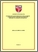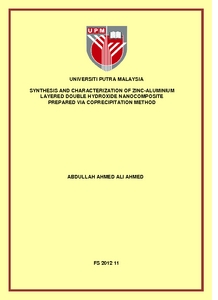# Synthesis and characterization of zinc-aluminium layered double hydroxide nanocomposite prepared via coprecipitation method

## Citation

Ali Ahmed, Abdullah Ahmed (2012) Synthesis and characterization of zinc-aluminium layered double hydroxide nanocomposite prepared via coprecipitation method. PhD thesis, Universiti Putra Malaysia.

## Abstract

Zinc–Aluminium Layered Double Hydroxides (Zn–Al–NO3–LDHs) were prepared using the coprecipitation method at different Zn2+/Al3+ molar ratios (2, 3, 4, 5 and 6) with adjusting pH value at 7.5 and at different pH values (6, 7, 8, 9 and 10) with constant molar ratio of 4. The second part of the work is the calcination of Zn–Al–NO3–LDH sample prepared at Zn2+/Al3+ molar ratio of 4 and pH value of 7 at two temperature ranges; 50–500 °C and 200–1000 °C. The structural, textural, morphological, thermal and optical properties were studied for all the samples. In addition, the dielectric responses and ESR spectra were also investigated. Analysis of the XRD spectra of LDH showed that the crystallite size was in the range of 15–36 nm which confirmed that the LDH is a nanocomposite. This is corroborated by the results of unit cell parameters (a and c) which showed that the construction of LDH resulted in nanolayers. For the calcined LDH sample at 200 °C and above, the crystallite size were in the range of 42.7–51.2 nm which is attributed to the formation nanostructure of ZnO phase. For the samples prepared at different Zn2+/Al3+ molar ratios, the crystallinity of LDHs was found to improve as molar ratio decreased due to the distortion of the hydroxide sheet networks of the LDH crystal by the larger difference in ionic radii of Zn2+ and Al3+. On the other hand, the crystallinity of the samples prepared at different pH-values was improved as the pH-value increased due to the increasing of the precipitation of Zn2+ ions into the LDH slurry. For the calcination temperature below 200 °C, LDH structure was well preserved,ZnOstructure was well preserved, but started to collapse and transformed zinc oxide (ZnOstructure was well preserved, but started to collapse and transformed zinc oxide (ZnOstructure was well preserved, (ZnO)phase at 200°C and above due to the water loss and decomposition nitrate anions of LDH interlayer At600 °C and above ZnAl2O4 spinel was formed and the crystallinity of ZnO and ZnAl2O4 improved as the calcination temperature increased. Opticle band gap of the samples was calculated using Kubelka-Munk model. The band gaps arise due to the electronic direct transitions in the brucite-like layers and nitrate aions (NO3–). Band gaps were affected by the molar ratios used due to the formation of low crystalline phases (ZnO and ZnAl2O4). This affect was also observed by the variation of pH-value.Band gap decreased as the calcination temperature increased within the experimental temperature range of 200-1000°C. The decreased in band gap value was related to the improvement of the photocatalytic activity due to the increased of the ZnO crystal size. The dielectric respone of the LDH is believed to be caused by two charge carriers: the protons of the LDH water molecules and the NO3 interlayer anions which can be described by the anomolous low frequency dispersion with the second type of Universal Power Law. The dominance of ZnO dipoles and charge carrier (NO3 ions) in the dielectric relaxation increases with the increasing molar ratio. The dielectric respone for the LDH ZnOLDH calcined above 150 °C is similar to the dielectric response of ZnOLDH calcined above 150 °C is similar to the dielectric response of ZnOLDH calcined above 150 °C is similar to the dielectric response of ZnOLDH calcined above 150 °C is similar to the dielectric response of ZnOLDH calcined above 150 °C is similar to the dielectric response of ZnOLDH calcined above 150 °C is similar to the dielectric response of ZnOLDH calcined above 150 °C is similar to the dielectric response of ZnOLDH calcined above 150 °C is similar to the dielectric response of ZnOLDH calcined above 150 °C is similar to the dielectric response of ZnO ZnOLDH calcined above 150 °C is similar to the dielectric response of ZnOLDH calcined above 150 °C is similar to the dielectric response of ZnOLDH calcined above 150 °C is similar to the dielectric response of ZnOLDH calcined above 150 °C is similar to the dielectric response of ZnOLDH calcined above 150 °C is similar to the dielectric response of ZnOLDH calcined above 150 °C is similar to the dielectric response of ZnOLDH calcined above 150 °C is similar to the dielectric response of ZnOLDH calcined above 150 °C is similar to the dielectric response of ZnOLDH calcined above 150 °C is similar to the dielectric response of ZnOLDH calcined above 150 °C is similar to the dielectric response of ZnOLDH calcined above 150 °C is similar to the dielectric response of ZnOLDH calcined above 150 °C is similar to the dielectric response of ZnOLDH calcined above 150 °C is similar to the dielectric response of ZnOLDH calcined above 150 °C is similar to the dielectric response of ZnO, because of the presence of the ZnO phase in the LDH at this temperature range. The in situ electron spin resonance (ESR) spectra of fresh LDH obtained at room temperature up to 190 °C were studied. These spectra were due to the presence of nitrate anions in the LDH interlayer. However, at at calcination temperature of 200°C and above. ESR spectra (ex situ ESR spectra) were due to the oxygen vacancies of ZnO which was formed during thermal of LDH, AT 600°C and above,there is an interaction between the oxygen vacancies of ZnAI2O4 and the aluminium atom. The overlapped signal can be resolved into two signals from the ZnAI2O4 soinel and the ZnO phase at 1000°C. Thermal diffusivity of LDH as a function Thermal diffusivity of LDH as a function in situ temperatures result in a nonlinear relation which is due to the changing of water content of LDH when the temperature was increased. However, thermal diffiusitivy thermal diffusivity of LDH as a function thermal diffusivity of LDH as a function thermal diffusivity of LDH as a function thermal diffusivity of LDH as a function thermal diffusivity of LDH as a function thermal diffusivity of LDH as a function calcined temperatures showed a linear relation and the slope of these data demonstrated the dependency between thermal diffusivity and water content of LDH below 200°C.For temperatures above 180 °C, the thermal diffusivity behavior was mainly governed by ZnO phase in the LDH. Themal diffisivity of LDH as a function of molar ratio also resulted in a nonlinear relation which is due to the formation other phases namely ZnO and ZnAl2O4 with LDH, when the molar ratio was decreased or pH-value was increased.Preview
PDF
FS 2012 11R.pdfView Item